### IMO Shortlist 2012 problem G5

Kvaliteta:
Avg: 3,0
Težina:
Avg: 8,0
Let$ABC$ be a triangle with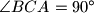$\angle BCA=90^{\circ}$, and let$D$ be the foot of the altitude from$C$. Let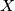$X$ be a point in the interior of the segment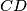$CD$. Let$K$ be the point on the segment$AX$ such that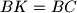$BK=BC$. Similarly, let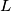$L$ be the point on the segment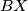$BX$ such that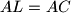$AL=AC$. Let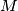$M$ be the point of intersection of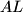$AL$ and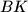$BK$.

Show that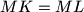$MK=ML$.

Proposed by Josef Tkadlec, Czech Republic
Izvor: Međunarodna matematička olimpijada, shortlist 2012